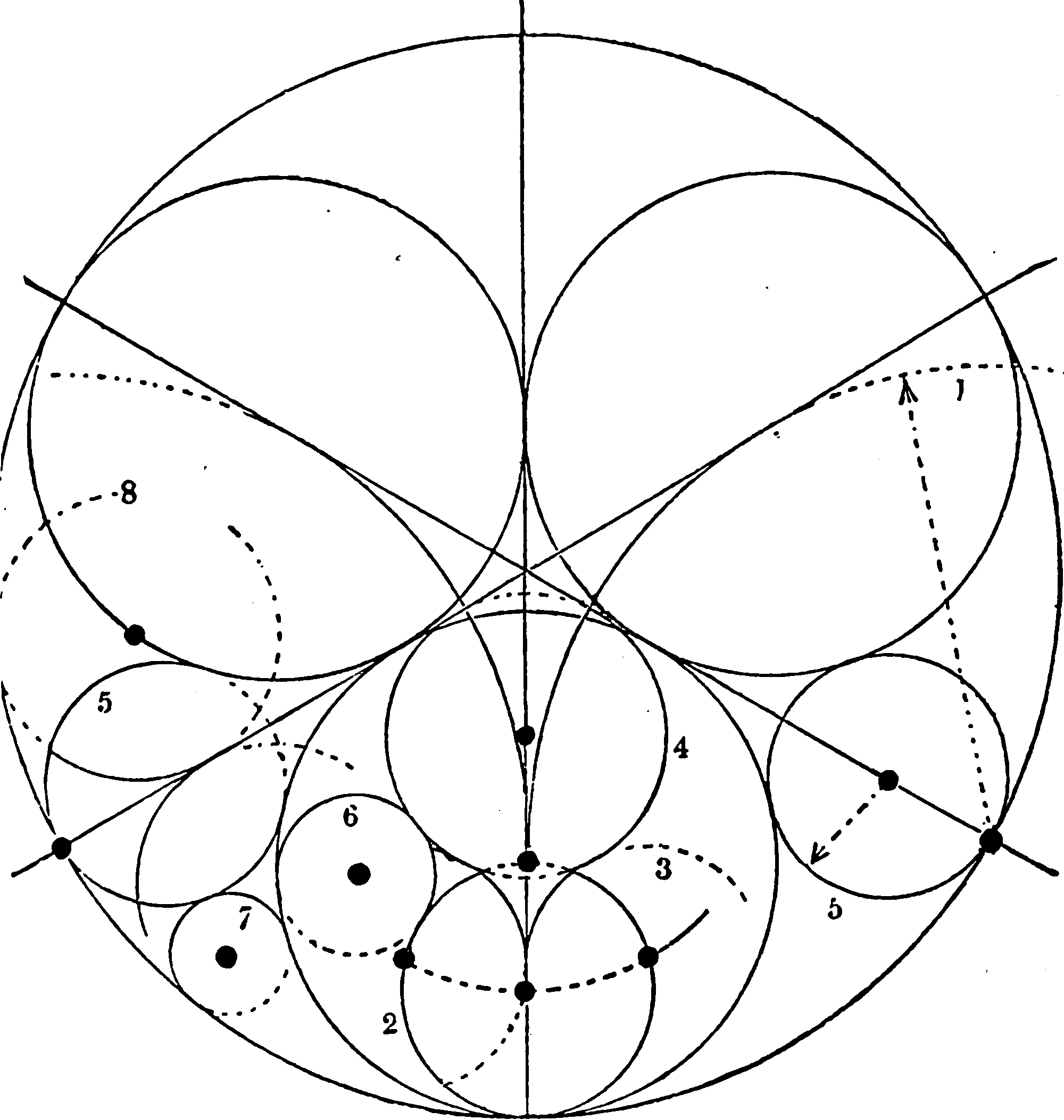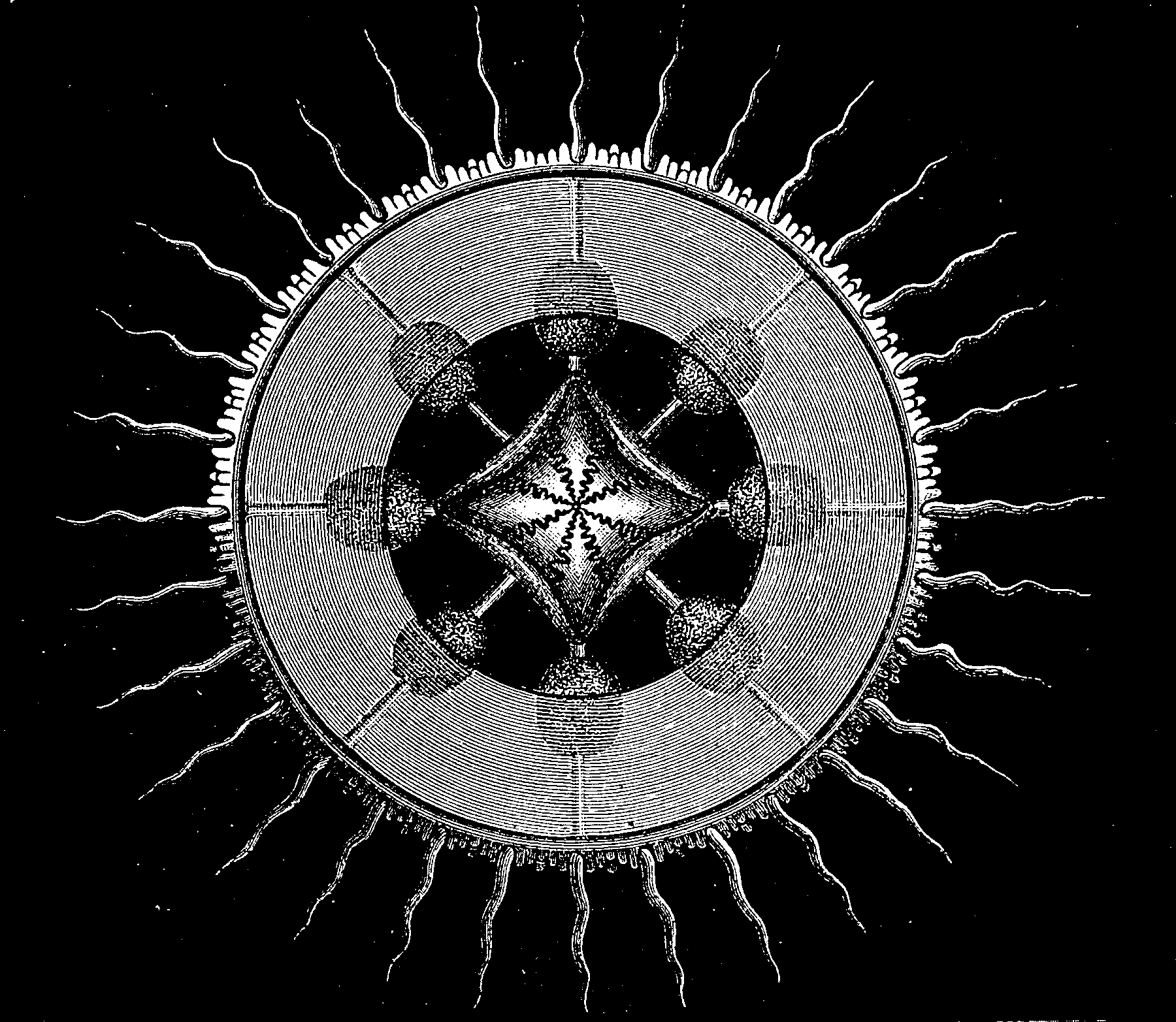# Fourier transformsPlaceholder.

The greatest of the integral transforms.

## Incoming## Useful properties

$$f(x)\,$$
$$\hat{f}(\nu) = \int f(x) e^{-i \nu x}\, dx$$
Linearity: $$a\cdot f(x) + b\cdot g(x)\,$$
$$a\cdot \hat{f}(\nu) + b\cdot \hat{g}(\nu)\,$$
Time shift $$f(x - a)\,$$
$$e^{- i a \nu} \hat{f}(\nu)\,$$
Frequency shift $$f(x)e^{iax}\,$$
$$\hat{f}(\nu - a)\,$$
Dilation $$f(a x)\,$$
$$\frac{1}{|a|} \hat{f}\left( \frac{\nu}{a} \right)\,$$
Duality $$\hat{f}(x)\,$$
$$2\pi f(-\nu)\,$$
$$\frac{d^n f(x)}{dx^n}\,$$
$$(i\nu)^n \hat{f}(\nu)\,$$
$$x^n f(x)\,$$
$$i^n \frac{d^n \hat{f}(\nu)}{d\nu^n}$$
Convolution $$(f * g)(x)\,$$
$$\hat{f}(\nu) \hat{g}(\nu)\,$$
$$f(x) g(x)\,$$
$$\frac{1}{2\pi}\left(\hat{f} * \hat{g}\right)(\nu)\,$$
Hermitian symmetry. For $$f(x)$$ purely real
$$\hat{f}(-\nu) = \overline{\hat{f}(\nu)}\,$$
For $$f(x)$$ purely real and even
$$f(\nu)$$ is purely real even functions.
For $$f(x)$$ purely real and odd
$$f(\nu)$$ are purely imaginary and odd.
For $$f(x)$$ purely imaginary
$$\hat{f}(-\nu) = -\overline{\hat{f}(\nu)}\,$$
Complex conjugation $$\overline{f(x)}$$
$$\overline{\hat{f}(-\nu)}$$
$$f(x) \cos (a x)$$
$$\frac{\hat{f}(\nu-a)+\hat{f}(\nu+a)}{2}$$
$$f(x)\sin( ax)$$
$$\frac{\hat{f}(\nu-a)-\hat{f}(\nu+a)}{2i}$$
$$e^{-\alpha x^{2}}$$
$${\sqrt{\frac {\pi }{\alpha }}}\cdot e^{-{\frac {\nu ^{2}}{4\alpha}}}$$
$$e^{-i\alpha x^{2}}$$
$${\sqrt{\frac {\pi }{\alpha }}} \cdot e^{i(\frac {\nu ^{2}}{4\alpha}-\frac {\pi }{4})}$$
$$f(x)e^{-\alpha x^{2}}$$
…anything useful?

## Interpolation

Fourier transforms are useful for cheap interpolations, specifically a magical kind of polynomial basis function.

## Enveloped Fourier transformsIf you multiply your function by an envelope or tapering function or window or whatever before taking a Fourier transform, then this is no longer strictly FT; but I wonder which features we can relate this back to FTs?

This is heavily studied in the context of time frequency analyses, but I have not found a reference for all the results I need, so I will derive a couple of my own here.

### No comments yet. Why not leave one?

GitHub-flavored Markdown & a sane subset of HTML is supported.